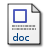# Negative real interest rates

Chen, Jing, Ma, Diandian, Song, Xiaojing and Tippett, Mark (2017) Negative real interest rates. The European Journal of Finance, 23 (15). pp. 1447-1467. ISSN 1351-847XMicrosoft Word (Negative real interest rates) - Accepted Version Download (530kB)

## Abstract

Standard textbook general equilibrium term structure models such as that developed by Cox, Ingersoll, and Ross [1985b. “A Theory of the Term Structure of Interest Rates.” Econometrica 53 (2): 385–407], do not accommodate negative real interest rates. Given this, the Cox, Ingersoll, and Ross [1985b. “A Theory of the Term Structure of Interest Rates.” Econometrica 53 (2): 385–407] ‘technological uncertainty variable’ is formulated in terms of the Pearson Type IV probability density. The Pearson Type IV encompasses mean-reverting sample paths, time-varying volatility and also allows for negative real interest rates. The Fokker–Planck (i.e. the Chapman–Kolmogorov) equation is then used to determine the conditional moments of the instantaneous real rate of interest. These enable one to determine the mean and variance of the accumulated (i.e. integrated) real rate of interest on a bank (or loan) account when interest accumulates at the instantaneous real rate of interest defined by the Pearson Type IV probability density. A pricing formula for pure discount bonds is also developed. Our empirical analysis of short-dated Treasury bills shows that real interest rates in the UK and the USA are strongly compatible with a general equilibrium term structure model based on the Pearson Type IV probability density.

Item Type: Article fokker–planck equation,mean reversion,real interest rate,pearson type iv probability density,c61,c63,e43 Faculty of Social Sciences > Norwich Business School Faculty of Social Sciences > Research Groups > Accounting, Finance and Governance (former - to 2017) http://www.tandfonline.com/doi/full/10.1... Pure Connector 22 Apr 2016 10:00 22 Oct 2022 01:02 https://ueaeprints.uea.ac.uk/id/eprint/58340 10.1080/1351847X.2016.1158729View Item